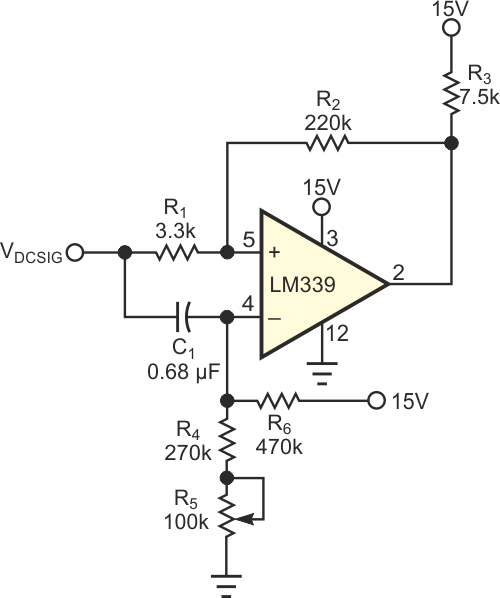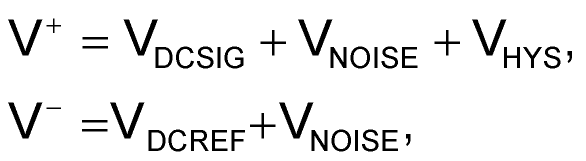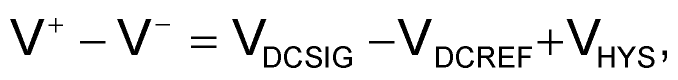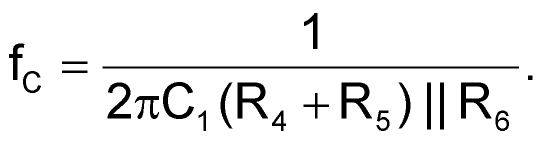# Filter allows comparison of noisy signals

## Intersil LM339

When you need to compare the dc level of a noisy signal with a reference for further processing, the output of the comparator changes in a chaotic way when the dc level approaches that of the reference. You have a choice of two classic solutions to this problem: One is to add hysteresis to the comparator, but, if the noise level is high, the hysteresis must be correspondingly high. In this situation, you face a wide dead-band zone around the comparison trip point. The second solution is to add lowpass filtering to the noisy signal.Figure 1. By presenting the noise signal to both inputs of the comparator, this circuit can compare the dc levels of the signal and the reference.

This approach increases the response time, slowing down the system. This Design Idea proposes a third solution that avoids the cited drawbacks. In the circuit in Figure 1, the noise adds to the reference through a highpass filter, so the comparator's inputs see only the difference between the two dc levels:andwhere V+ is the voltage on the comparator's positive input, Vis the voltage on the negative input, VNOISE is the noise riding on the signal, and VHYS is the hysteresis accruing from the positive feedback to the positive input.

C1, R4, R5, and R6 form the highpass filter, whose cutoff frequency isThe cutoff frequency should be lower than the lowest frequency of the noise band. R1 and R2 establish the still-needed small amount of hysteresis. R3 is a pullup resistor for the open-collector output of the comparator. The comparator circuit worked successfully in a system that processes the fluctuating current generated by an ionization chamber in a neutron-flux measurement system.

## Materials on the topic

EDN